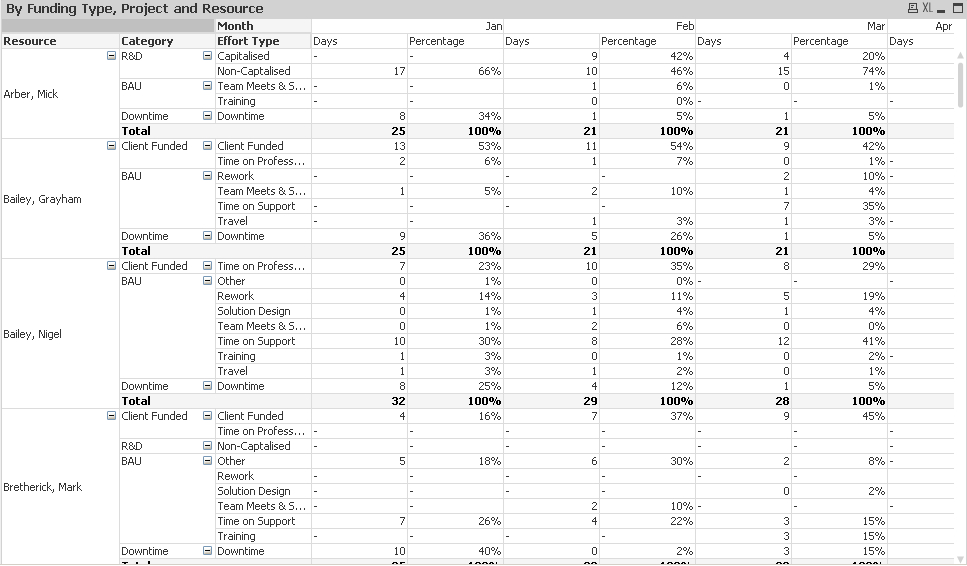# QlikView App Development

Discussion Board for collaboration related to QlikView App Development.Not applicable

## Help calculating percentages in a pivot

Hi all,

I attach a qvw where I am trying to calculate percentages within the pivot.

For example, the pivot is by resource, category and effort type - and then by month

showing a total number of days/

what I need to be able to do is see a percentage for each month, for each resource of the time by effort type.

so for each month, the numbers add up to 100% for each resource

I'm stumped though - playing with aggr function, but doing it wrong.

help would be appreciated

many thanks,

Matt

1 Solution

Accepted Solutions
Contributor III

## Re: Help calculating percentages in a pivot

You can use both dimensions for total qualifier =SUM(HOURS)/SUM(total <Month, FULL_NAME> HOURS) or, as many as you need.5 Replies
Highlighted
Contributor III

## Re: Help calculating percentages in a pivot

Hello Matthew,

You can use an expression like =SUM(HOURS)/SUM(total <Month> HOURS).

Regards,

DavidNot applicable

## Re: Help calculating percentages in a pivot

Hi David,

thanks for your response.

That calculates percentage across the WHOLE pivot - with all entries adding up to 100

I want each Resource (FULL_NAME field) to add up to a 100, but also each month within that

any ideas?Not applicable

## Re: Help calculating percentages in a pivot

if I take your expression =SUM(HOURS)/SUM(total <Month> HOURS).

and change Month to 'FULL_NAME'

=SUM(HOURS)/SUM(total <FULL_NAME> HOURS)

it gives me on a total level the correct sort of thing.

But  each entry for a resource is a percentage of all months.

Contributor III

## Re: Help calculating percentages in a pivot

You can use both dimensions for total qualifier =SUM(HOURS)/SUM(total <Month, FULL_NAME> HOURS) or, as many as you need.Not applicable

## Re: Help calculating percentages in a pivot

Thansk David.

out of interest, what is that 'function' called and how does it differ from AGGR?

Matt